# ISEE Lower Level Quantitative : How to find the area of a parallelogram

## Example Questions

1 2 12 13 14 15 16 17 18 20 Next →

### Example Question #12 : Solving For Area

Joe has a piece of wallpaper that is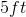by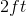. How much of a wall can be covered by this piece of wallpaper?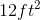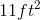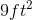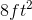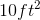Explanation:

This problem asks us to calculate the amount of space that the wallpaper will cover. The amount of space that something covers can be described as its area. In this case area is calculated by using the formula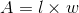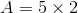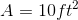### Example Question #281 : Measurement & Data

Joe has a piece of wallpaper that is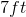by. How much of a wall can be covered by this piece of wallpaper?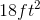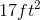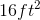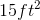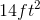Explanation:

This problem asks us to calculate the amount of space that the wallpaper will cover. The amount of space that something covers can be described as its area. In this case area is calculated by using the formula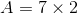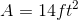### Example Question #281 : Measurement & Data

Joe has a piece of wallpaper that is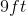by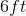. How much of a wall can be covered by this piece of wallpaper?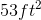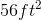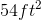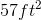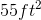Explanation:

This problem asks us to calculate the amount of space that the wallpaper will cover. The amount of space that something covers can be described as its area. In this case area is calculated by using the formula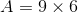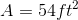### Example Question #121 : Rectangles

Joe has a piece of wallpaper that isby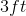. How much of a wall can be covered by this piece of wallpaper?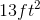Explanation:

This problem asks us to calculate the amount of space that the wallpaper will cover. The amount of space that something covers can be described as its area. In this case area is calculated by using the formula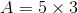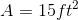### Example Question #122 : Rectangles

Joe has a piece of wallpaper that isby. How much of a wall can be covered by this piece of wallpaper?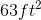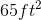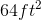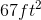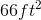Explanation:

This problem asks us to calculate the amount of space that the wallpaper will cover. The amount of space that something covers can be described as its area. In this case area is calculated by using the formula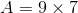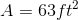### Example Question #191 : How To Find The Area Of A Parallelogram

What is the length of a rectangular room with a perimeter of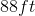and a width of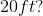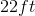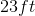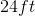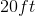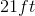Explanation: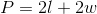We have the perimeter and the width, so we can plug those values into our equation and solve for our unknown.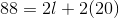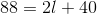Subtractfrom both sides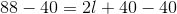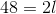Divideby both sides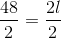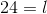1 2 12 13 14 15 16 17 18 20 Next →

### All ISEE Lower Level Quantitative Resources